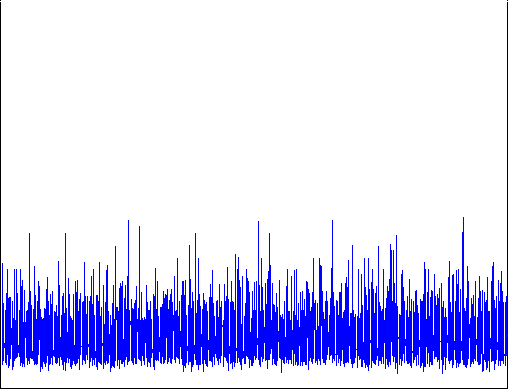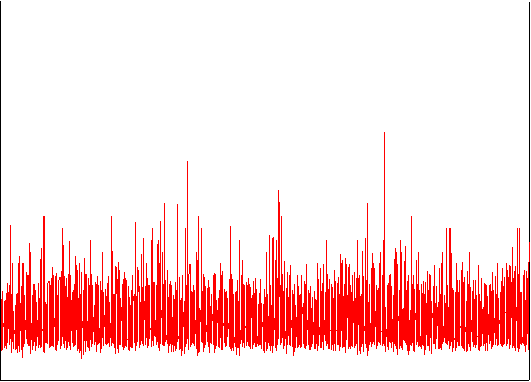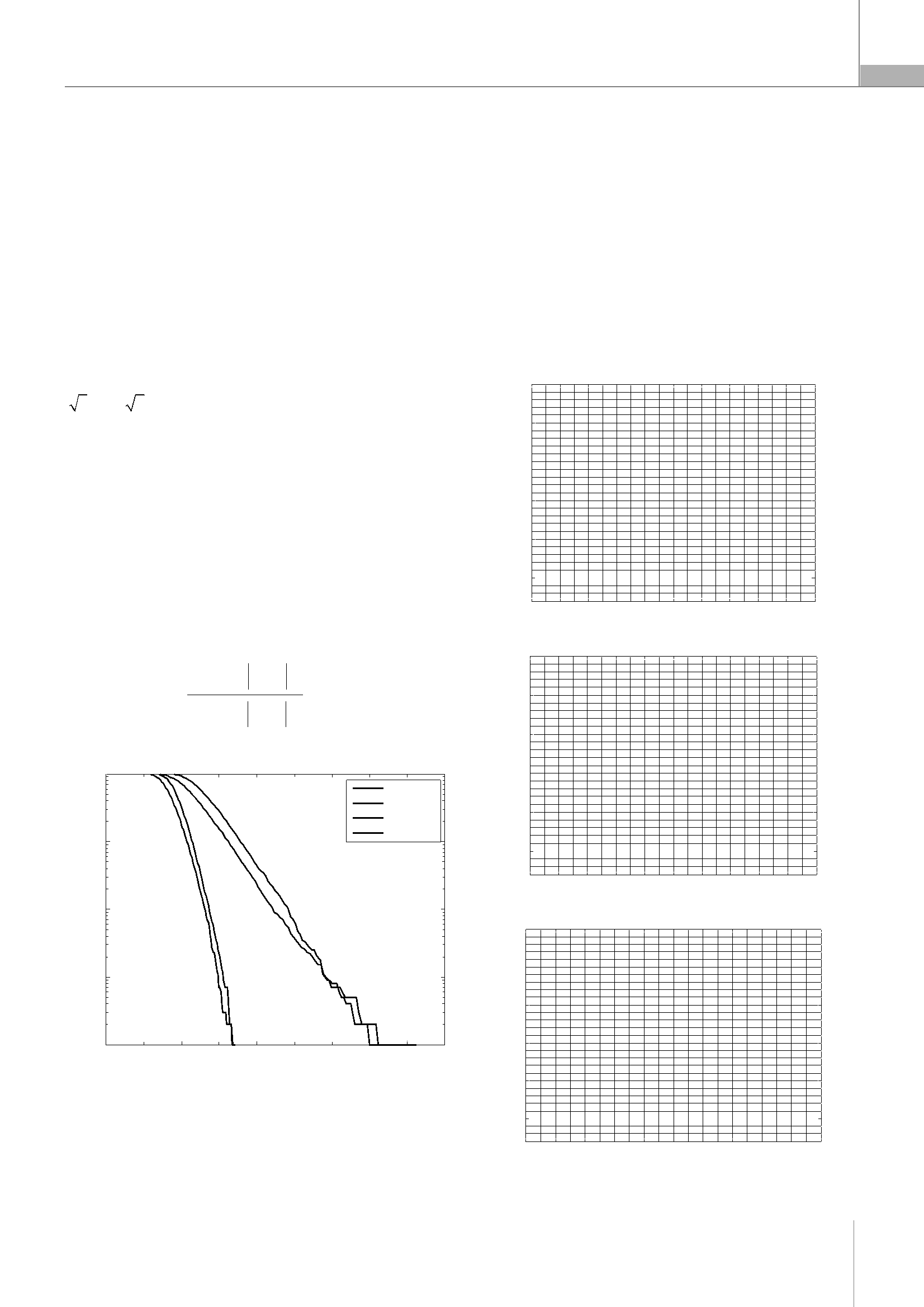SCIENCE TECHNOLOGY
PERFORMANCE EVALUATION OF CI-VOFDM
FOR PAPR REDUCING
ĐÁNH GIÁ HIỆU NĂNG HỆ THỐNG CI-VOFDM ĐỂ GIẢM TỶ SỐ CÔNG SUẤT ĐỈNH TRUNG BÌNH
Nguyễn Thị Diệu Linh1,*,
Phan Thị Thu Hằng1, Trần Ngọc Đức2
ABSTRACT
High Pick to Average Power Ratio (PAPR) contributes to increasing in
complexity of the Analog to Digital Converter (ADC) and the Digital to Analog
Converter (DAC), as a result, it reduced efficiency of the High Power Amplifier
(HPA) and degradation of orthogonal frequency division multiplexing (OFDM)
system performance. In this paper, the carrier interferometry (CI) spreading code
in VectorOFDM (V-OFDM)to reduce the PAPR value is proposed. The simulation
shows that the PAPR value decreases in CI-V OFDM systems compare to the
conventional OFDM system.
Keywords: Pick to Average Power Ratio (PAPR), Carrier Interferometry (CI),
Orthogonal Frequency Division Multiplexing (OFDM).
peak power pass through an HPA, high Out of Band (OOB)
distortions may occur . To prevent saturation and
clipping of OFDM signal peaks, the power amplifier must
not be driven to saturation, i.e., the amplifier operates with
sufficient Back-Off (BO) that will increase with the PAPR.
The power fluctuations can be measured by Peak to
Average Power Ratio (PAPR), Crest Factor (CF), and Power
Ratio (PR). High PAPR causes drawbacks like an increased
complexity of Analog to Digital Converter (ADC) and Digital
to Analog Converter (DAC), a reduced efficiency of the HPA
High PAPR demands the DAC/ADC with enough
TÓMTẮT
dynamic range to accommodate the high peaks of the
Tỷ lệ công suất đỉnh trung bình cao (PAPR) là nguyên nhân làm tăng độ
phức tạp của bộ chuyển đổi tương tự số (ADC) và chuyển đổi số sang tương tự
(DAC), làm giảm hiệu quả của bộ khuếch đại phát (HPA) và giảm hiệu suất của hệ
thống ghép đa tần trực giao theo tần số OFDM. Bài báo này đề cập đến giải pháp
OFDM signals High PAPR requires a high precision DAC
with a reasonable amount of quantization noise which
might be very expensive for a given sampling rate of the
system .
mã lan truyền chéo (CI) trong VOFDM nhằm giảm giá trị công suất đỉnh trung
Also high PAPR will require an HPA with wide dynamic
bình. Kết quả mô phỏng cho thấy giá trị của PAPR trong hệ thống CI-VOFDM
range to operate in linear region, i.e., HPA needs a back-off
giảm so với hệ thống OFDM thông thường.
to make sure it remain linear over an amplitude range that
Từ khóa: Tỷ lệ công suất đỉnh trung bình cao, lan truyền chéo, ghép đa tần
trực giao theo tần số.
include the high peak amplitudes. The expansion of the
linear region increases the cost of the power amplifier.
Nonlinearity characteristics cause spectral widening of
1Khoa Điện tử, Trường Đại học Công nghiệp HàNội
2Khoa Điện - Điện tử, Trường Đại học Sư phạm Kỹ thuật Nam Định
*Email: dieulinh79@gmail.com
Ngày nhận bài: 04/01/2018
Ngày nhận bài sửa sau phản biện: 02/4/2018
Ngày chấp nhận đăng: 21/8/2018
Phản biện kha học: TS. Dư Đình Viên
transmitting signal resulting in ISI and Inter-Channel
Interference (ICI) which degrades the system performance.
Diminishing the PAPR value has been proposed by
several methods, which basically can be classified into
three types. First, the signal distortion techniques reduce
the peak amplitudes by nonlinearly distorting the OFDM
signal at or around the peaks. This category includes the
clipping, peak windowing and peak cancellation
techniques. Second, the coding techniques use a special
forward error correcting code to exclude OFDM symbols
1. INTRODUCTION
The OFDM system divides the high data rate sequence
into parallel low data rate sequence, where the low data
rate symbols are simultaneously transmitted through the
orthogonal subcarriers. The transmission of each symbol on
its unique carrier leads to the potential for high peak power
because there is a possibility of all in phase bits on
subcarriers to add up coherently. When signals with high
with large PAPR value. Third, scrambling techniques
scrambles each OFDM symbol with different scrambling
sequences and selecting the sequence that provides the
lowest PAPR value.
This paper proposed the carrier interferometry (CI)
spreading code to be introduced in the precoded/vector
OFDM to combat the high PAPR. The CI code lies under the
second category of reducing PAPR in OFDM systems.
Số 48.2018Tạp chí KHOA HỌC & CÔNG NGHỆ
83k
N 1
1
x
2
 
 
 
 
 
 
2
x
2
X
2
x
x
2

k
'
k 0
k
 
2
k
 
 
 
k k
'
k
 
k
 
N
 
 
N
 
 
1 1
2 2
 
 
N N
k k
1
N
1
N
2
 
2
1
1
 
KHOA HỌC CÔNG NGHỆ
2. BRIEF REVIEW OF PEAK TO AVERAGE POWER RATIO
(PAPR)
Apart from the advantage of Precoded-OFDM and
Vector-OFDM (VOFDM) of being robust to ISI channel
spectral nulls and the reduction of cyclic prefix
transmission data rate, respectively, therePAPR value is not
convincible in comparison with the conventional OFDM
max x 2
PAPRmax N0kN1 2 (6)
N n0 k
In phase modulation schemes, the peak power and
average power have the same value and the PAPR is equal
to N.
system. In case of Carrier Interferometry OFDM (CI/OFDM),
The distribution function of PAPR is most commonly
the PAPR value is lower compared to the conventional
used
to
evaluate
the
performance
of
PAPR
reduction
OFDM system. This brought an idea of combining the
methods [3,4]. For large values of N typically greater or
Precoded-OFDM/VOFDM and CI/OFDM is resulting in the CI
equal to 64, real and imaginary parts of the transmitted
- VOFDM system. The PAPR of the transmitted OFDM signal
signal approach the Gaussiandistribution with a zero mean
x(n) is the ratio between the peak power value per OFDM
and a common variantx . However, it is better to consider
symbol and its average power value per OFDM symbol.
the distribution of the OFDM signal envelope because the
The PAPR of OFDM signal xnis the ratio of the
maximum power and its average power , mathematically
given by
PAPR depends on the signal envelope and the power has a
central chi-square distribution with two degrees of
freedom, the envelope is Rayleigh distributed. The
Cumulative Distribution Function (CDF) is given by
max x n 2
PAPR x n 0nN1
E xn
(1)
F x0 u expu2 2x du
=1-expx2 22 , x 0
(7)
Where the numerator of equation (1) expresses the
maximum power and the denominator is the average
power of the transmitted signal, Edenotes the
The probability that the PAPRxx22 is less than or
x
equal to a given threshold PAPR0 for Nsubcarriersis
expectation. The power of OFDM signal isdefined as:
x2 nN1 N1 xkx*' ej2nkk' N
N1 xk 0 N1xkx*' ej2nkk' N (2)
k0 k0 kk'
The peak power of OFDM signal isdefined as
max x n 2 max N1 x 2 N1 x x* ej2n kk'N
0nN1 0nN1k0 k0kk' (3)
N2max x 2
k
The relation between time and frequency domain
average power is
PPAPRPAPR0 CDFN (8)
= 1exp PAPR0
The probability that the PAPR is greater than PAPR0 is
defined as
PPAPR PAPR0 1CDFN (9)
=11exp PAPR0
Equation (8) and (9) defines the Complementary
Cumulative Distribution Function (CCDF), the statistical
method that provides the amount of time a signal spends
above any given power level. It can be seen that the
(P(PAPR > PAPR0) increases as the number of subcarriers
increases at any level.
N1 N1
xnxnN
n0 n0
(4)
3. PAPR REDUCTION METHODS
CI was proposed in  to eliminate the large power
peaks and, hence, reduce the PAPR in OFDM system
Substituting equation (3) and (4) into (1), the PAPR
equation becomes
N2 max x 2 max x 2
PAPRx0kN1 N0kN1 (5)
NN n0 xk N n0 xk
The inequality in the PAPR will turn to equality only if
the value of allxk’s is maximal. The maximum PAPR which is
the worst case is denoted by
without raising the system complexity. The evaluation of
system performance of MIMO STBC OFDM and MIMO STBC
CI/OFDM was introduced in [6,7]. The theoretical analysis
was studied when there is an existence of HPA nonlinearity
and the introduction of CI codes brought a big
improvement in the system.
A combination of CI spreading code and peak-
windowing with clipping/filtering of a single iteration was
proposed in [8-10]. The spreading was followed by peak-
windowing and clipping before transmission of the OFDM
84
Tạp chí KHOA HỌC & CÔNG NGHỆ Số 48.2018 
 
2
2
N 1
 
v
1
SCIENCE TECHNOLOGY
signal. The definition of efficient PAPR was defined as the
distribution
function
(CCDF)
and
PAPR
level
is
PAPR with minimum total degradation (TD), TD is the sum
demonstrated in thissection.
of signal to noise ratio of SSPA (SNRSSPA) and the OBO of
SSPA (OBOSSPA) in dB. There was an improvement in the
reduction of PAPR and no spectrum broadening due to the
clipping process.
Using N = 1024, V = M = 2, and QPSK modulation figure
1 shows the CCDF of conventional OFDM, VOFDM,
CI/OFDM, and CI-VOFDM systems, 10 000 data symbols are
generated.
The rotated precoder was proposed to reduce PAPR
in the precoded-OFDM system with zero padding half of
the information symbols forN -point FFT. Description of
From figure 1, CI-OFDM described the gain of about 8
dB compared to VOFDM and it is comparable to that of the
CI/OFDM system.
rotated precoder based OFDM was mentioned in chapter
two. The wavelet transformation was proposed as the
precoder to perform decomposition over the vector
information symbols from the binary phase shift keying
(BPSK) modulator. The half of the information symbols are
With N = 256 carriers across 10000 transmissions under
the conventional OFDM, precoded-OFDM, VOFDM, CI/OFDM
and CI-VOFDMsystem, we have the following results:
(a) OFDM PAPR per Transmission
30
2 or
2 and the remaining half of the information
symbols are zeros[12,13].
25
In
CI-VOFDM,
each
vector
sequence
is
transmitted
simultaneously over all carriers and the phase offset makes
20
symbols separable at the receiver. Also the phase offset
makes the symbols notto add coherently at the same time,
15
i.e. when one symbol’s add coherently, the others do not.
The peak power of CI-VOFDM is lower than that of OFDM
10
(sum of peak carrier powers), since when the power of one
symbol
slreaches
the
peak
power,
symbol
sb
is
at
5
minimum power. The average power of CI-VOFDM is equal
to OFDM average power. The PAPR of CI-VOFDM in the
0
1000
2000
3000 4000 5000 6000 7000
Transmission number
8000
9000 10000
worst case is given by:
(b) Precoded-OFDM PAPR per Transmission
1 max sv n 2
PAPRworstcase 0nN1 N
N n0 s n
(10)
30
25
4. SIMULATUON RESULTS
20
100
15
OFDM
VOFDM
CI/OFDM
10
10-1
CI-VOFDM
5
0
1000
2000
3000
4000
5000
6000
7000
8000
9000 10000
Transmission number
10-2
(c) VOFDM PAPR per Transmission
30
10-3
25
20
10-42
4
6
8
10
12
14
16
18
20
15
PAPR0
Figure 1. Complementary Cumulative distribution functions for conventional
10
OFDM, VOFDM, CI/OFDM, and CI- VOFDM systems
Assuming that the modulation and demodulation are
5
ideal QPSK. The comparison of rotated precoder based
OFDM and CI-VOFDM complementary cumulative
0
1000
2000
3000 4000 5000 6000 7000
Transmission number
8000
9000 10000
Số 48.2018Tạp chí KHOA HỌC & CÔNG NGHỆ
85
Pr(PAPR>PAPR0)
PAPR
PAPR
PAPR